Warning

This documents an unmaintained version of NetworkX. Please upgrade to a maintained version and see the current NetworkX documentation.

# Graph generators¶

## Atlas¶

Generators for the small graph atlas.

See “An Atlas of Graphs” by Ronald C. Read and Robin J. Wilson, Oxford University Press, 1998.

Because of its size, this module is not imported by default.

 graph_atlas_g() Return the list [G0,G1,...,G1252] of graphs as named in the Graph Atlas.

## Classic¶

Generators for some classic graphs.

The typical graph generator is called as follows:

>>> G=nx.complete_graph(100)


returning the complete graph on n nodes labeled 0,..,99 as a simple graph. Except for empty_graph, all the generators in this module return a Graph class (i.e. a simple, undirected graph).

 balanced_tree(r, h[, create_using]) Return the perfectly balanced r-tree of height h. barbell_graph(m1, m2[, create_using]) Return the Barbell Graph: two complete graphs connected by a path. complete_graph(n[, create_using]) Return the complete graph K_n with n nodes. complete_multipartite_graph(*block_sizes) Returns the complete multipartite graph with the specified block sizes. circular_ladder_graph(n[, create_using]) Return the circular ladder graph CL_n of length n. cycle_graph(n[, create_using]) Return the cycle graph C_n over n nodes. dorogovtsev_goltsev_mendes_graph(n[, ...]) Return the hierarchically constructed Dorogovtsev-Goltsev-Mendes graph. empty_graph([n, create_using]) Return the empty graph with n nodes and zero edges. grid_2d_graph(m, n[, periodic, create_using]) Return the 2d grid graph of mxn nodes, each connected to its nearest neighbors. grid_graph(dim[, periodic]) Return the n-dimensional grid graph. hypercube_graph(n) Return the n-dimensional hypercube. ladder_graph(n[, create_using]) Return the Ladder graph of length n. lollipop_graph(m, n[, create_using]) Return the Lollipop Graph;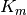connected to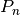. null_graph([create_using]) Return the Null graph with no nodes or edges. path_graph(n[, create_using]) Return the Path graph P_n of n nodes linearly connected by n-1 edges. star_graph(n[, create_using]) Return the Star graph with n+1 nodes: one center node, connected to n outer nodes. trivial_graph([create_using]) Return the Trivial graph with one node (with integer label 0) and no edges. wheel_graph(n[, create_using]) Return the wheel graph: a single hub node connected to each node of the (n-1)-node cycle graph.

## Expanders¶

Provides explicit constructions of expander graphs.

 margulis_gabber_galil_graph(n[, create_using]) Return the Margulis-Gabber-Galil undirected MultiGraph on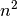nodes. chordal_cycle_graph(p[, create_using]) Return the chordal cycle graph on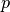nodes.

## Small¶

Various small and named graphs, together with some compact generators.

 make_small_graph(graph_description[, ...]) Return the small graph described by graph_description. LCF_graph(n, shift_list, repeats[, create_using]) Return the cubic graph specified in LCF notation. bull_graph([create_using]) Return the Bull graph. chvatal_graph([create_using]) Return the Chvátal graph. cubical_graph([create_using]) Return the 3-regular Platonic Cubical graph. desargues_graph([create_using]) Return the Desargues graph. diamond_graph([create_using]) Return the Diamond graph. dodecahedral_graph([create_using]) Return the Platonic Dodecahedral graph. frucht_graph([create_using]) Return the Frucht Graph. heawood_graph([create_using]) Return the Heawood graph, a (3,6) cage. house_graph([create_using]) Return the House graph (square with triangle on top). house_x_graph([create_using]) Return the House graph with a cross inside the house square. icosahedral_graph([create_using]) Return the Platonic Icosahedral graph. krackhardt_kite_graph([create_using]) Return the Krackhardt Kite Social Network. moebius_kantor_graph([create_using]) Return the Moebius-Kantor graph. octahedral_graph([create_using]) Return the Platonic Octahedral graph. pappus_graph() Return the Pappus graph. petersen_graph([create_using]) Return the Petersen graph. sedgewick_maze_graph([create_using]) Return a small maze with a cycle. tetrahedral_graph([create_using]) Return the 3-regular Platonic Tetrahedral graph. truncated_cube_graph([create_using]) Return the skeleton of the truncated cube. truncated_tetrahedron_graph([create_using]) Return the skeleton of the truncated Platonic tetrahedron. tutte_graph([create_using]) Return the Tutte graph.

## Random Graphs¶

Generators for random graphs.

 fast_gnp_random_graph(n, p[, seed, directed]) Returns a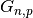random graph, also known as an Erdős-Rényi graph or a binomial graph. gnp_random_graph(n, p[, seed, directed]) Returns arandom graph, also known as an Erdős-Rényi graph or a binomial graph. dense_gnm_random_graph(n, m[, seed]) Returns a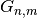random graph. gnm_random_graph(n, m[, seed, directed]) Returns arandom graph. erdos_renyi_graph(n, p[, seed, directed]) Returns arandom graph, also known as an Erdős-Rényi graph or a binomial graph. binomial_graph(n, p[, seed, directed]) Returns arandom graph, also known as an Erdős-Rényi graph or a binomial graph. newman_watts_strogatz_graph(n, k, p[, seed]) Return a Newman–Watts–Strogatz small-world graph. watts_strogatz_graph(n, k, p[, seed]) Return a Watts–Strogatz small-world graph. connected_watts_strogatz_graph(n, k, p[, ...]) Returns a connected Watts–Strogatz small-world graph. random_regular_graph(d, n[, seed]) Returns a random d-regular graph on n nodes. barabasi_albert_graph(n, m[, seed]) Returns a random graph according to the Barabási–Albert preferential attachment model. powerlaw_cluster_graph(n, m, p[, seed]) Holme and Kim algorithm for growing graphs with powerlaw degree distribution and approximate average clustering. duplication_divergence_graph(n, p[, seed]) Returns an undirected graph using the duplication-divergence model. random_lobster(n, p1, p2[, seed]) Returns a random lobster graph. random_shell_graph(constructor[, seed]) Returns a random shell graph for the constructor given. random_powerlaw_tree(n[, gamma, seed, tries]) Returns a tree with a power law degree distribution. random_powerlaw_tree_sequence(n[, gamma, ...]) Returns a degree sequence for a tree with a power law distribution.

## Degree Sequence¶

Generate graphs with a given degree sequence or expected degree sequence.

 configuration_model(deg_sequence[, ...]) Return a random graph with the given degree sequence. directed_configuration_model(...[, ...]) Return a directed_random graph with the given degree sequences. expected_degree_graph(w[, seed, selfloops]) Return a random graph with given expected degrees. havel_hakimi_graph(deg_sequence[, create_using]) Return a simple graph with given degree sequence constructed using the Havel-Hakimi algorithm. directed_havel_hakimi_graph(in_deg_sequence, ...) Return a directed graph with the given degree sequences. degree_sequence_tree(deg_sequence[, ...]) Make a tree for the given degree sequence. random_degree_sequence_graph(sequence[, ...]) Return a simple random graph with the given degree sequence.

## Random Clustered¶

Generate graphs with given degree and triangle sequence.

 random_clustered_graph(joint_degree_sequence) Generate a random graph with the given joint independent edge degree and triangle degree sequence.

## Directed¶

Generators for some directed graphs, including growing network (GN) graphs and scale-free graphs.

 gn_graph(n[, kernel, create_using, seed]) Return the growing network (GN) digraph with n nodes. gnr_graph(n, p[, create_using, seed]) Return the growing network with redirection (GNR) digraph with n nodes and redirection probability p. gnc_graph(n[, create_using, seed]) Return the growing network with copying (GNC) digraph with n nodes. scale_free_graph(n[, alpha, beta, gamma, ...]) Returns a scale-free directed graph.

## Geometric¶

Generators for geometric graphs.

 random_geometric_graph(n, radius[, dim, pos]) Returns a random geometric graph in the unit cube. geographical_threshold_graph(n, theta[, ...]) Returns a geographical threshold graph. waxman_graph(n[, alpha, beta, L, domain]) Return a Waxman random graph. navigable_small_world_graph(n[, p, q, r, ...]) Return a navigable small-world graph.

## Line Graph¶

Functions for generating line graphs.

 line_graph(G[, create_using]) Returns the line graph of the graph or digraph G.

## Ego Graph¶

Ego graph.

 ego_graph(G, n[, radius, center, ...]) Returns induced subgraph of neighbors centered at node n within a given radius.

## Stochastic¶

Functions for generating stochastic graphs from a given weighted directed graph.

 stochastic_graph(G[, copy, weight]) Returns a right-stochastic representation of the directed graph G.

## Intersection¶

Generators for random intersection graphs.

 uniform_random_intersection_graph(n, m, p[, ...]) Return a uniform random intersection graph. k_random_intersection_graph(n, m, k) Return a intersection graph with randomly chosen attribute sets for each node that are of equal size (k). general_random_intersection_graph(n, m, p) Return a random intersection graph with independent probabilities for connections between node and attribute sets.

## Social Networks¶

Famous social networks.

 karate_club_graph() Return Zachary’s Karate Club graph. davis_southern_women_graph() Return Davis Southern women social network. florentine_families_graph() Return Florentine families graph.

## Community¶

Generators for classes of graphs used in studying social networks.

 caveman_graph(l, k) Returns a caveman graph of l cliques of size k. connected_caveman_graph(l, k) Returns a connected caveman graph of l cliques of size k. relaxed_caveman_graph(l, k, p[, seed]) Return a relaxed caveman graph. random_partition_graph(sizes, p_in, p_out[, ...]) Return the random partition graph with a partition of sizes. planted_partition_graph(l, k, p_in, p_out[, ...]) Return the planted l-partition graph. gaussian_random_partition_graph(n, s, v, ...) Generate a Gaussian random partition graph.

## Non Isomorphic Trees¶

Implementation of the Wright, Richmond, Odlyzko and McKay (WROM) algorithm for the enumeration of all non-isomorphic free trees of a given order. Rooted trees are represented by level sequences, i.e., lists in which the i-th element specifies the distance of vertex i to the root.

 nonisomorphic_trees(order[, create]) Returns a list of nonisomporphic trees number_of_nonisomorphic_trees(order) Returns the number of nonisomorphic trees